﻿ 手动变速器换挡操作机构频响分析

# 手动变速器换挡操作机构频响分析Frequency Response Analysis of Manual Transmission Shifting Operation Mechanism

Abstract: Aiming at the problem that the secondary impact caused by shifting of a domestic vehicle manual transmission affects the quality of shifting operation of users, the performance of the external con-trol mechanism of gearbox was studied and its structural dynamic response performance was opti-mized to reduce the secondary impact of shifting. By creating the finite element model of the exter-nal control mechanism of the manual transmission and using NASTRAN analysis tool, the frequency response analysis and demonstration are carried out based on the calculation with theoretical deri-vation of mode and frequency response. The experimental results show that the simulation results are accurate and reliable. Finally, the factors that affected the secondary impact were discussed from the system damping coefficient and the stiffness of the cable bracket. The results show that increasing the damping of the shift system, increasing the stiffness of the shift cable bracket and reducing the stiffness of the shift cable joint were beneficial to reducing the secondary impact of the shift.

1. 引言

2. 换挡机构分析

2.1. 换挡机构工作原理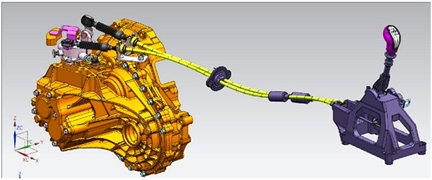Figure 1. Shift out operating mechanism

2.2. 换挡机构模态

$\left[M\right]\left\{\stackrel{¨}{x}\right\}+\left[C\right]\left\{\stackrel{˙}{x}\right\}+\left[K\right]\left\{x\right\}=\left\{f\right\}$ (1)

$\left[H\left(s\right)\right]=\frac{\left\{X\left(s\right)\right\}}{\left\{F\left(s\right)\right\}}={\left[Z\left(s\right)\right]}^{-1}=\frac{1}{{s}^{2}\left[M\right]+s\left[C\right]+\left[K\right]}$ (2)

2.3. 换挡机构频响分析理论

$m\stackrel{¨}{x}+c\stackrel{˙}{x}+kx={P}_{0}\mathrm{sin}\omega t$ (3)

$x=B\mathrm{sin}\left(\omega t-\varphi \right)$ (4)

$H\left(\omega \right)=\frac{x\left(w\right)}{P\left(w\right)}=\frac{1}{k-m{\omega }^{2}+ic\omega }$ (5)

$A\left(\omega \right)=\frac{\frac{1}{{\omega }_{n}^{2}}}{\sqrt{{\left[1-{\left(\frac{\omega }{{\omega }_{n}}\right)}^{2}\right]}^{2}+{\left[2\xi \frac{\omega }{{\omega }_{n}}\right]}^{2}}}$ (6)Figure 2. Amplitude frequency diagram

3. 有限元模型的建立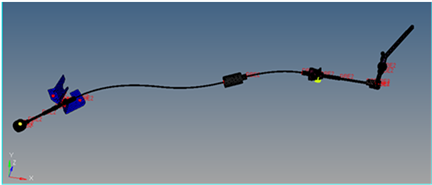Figure 3. Fem model of external control systemTable 1. Material name and parameter list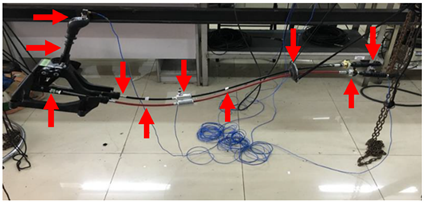Figure 4. Select the location of the test pointsTable 2. Comparative analysis of free modal simulation and experimental results

4. 频率响应分析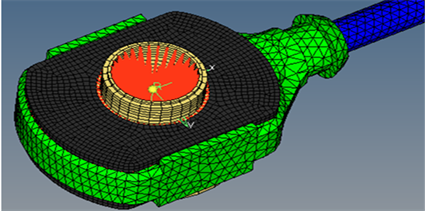Figure 5. The position where dynamic loads are applied

4.1. 系统阻尼系数对振动响应的影响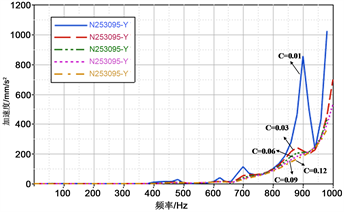(a)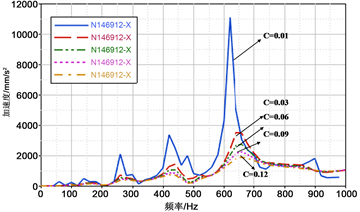(b)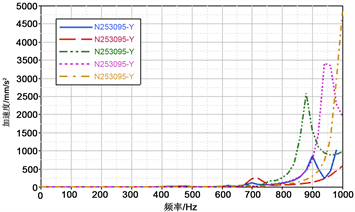(c)

Figure 6. Response of corresponding position under different damping coefficients. (a) End of the rod; (b) Balancing weight; (c) Shift stay

4.2. 拉索支架刚度对振动响应的影响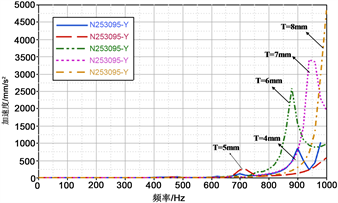(a)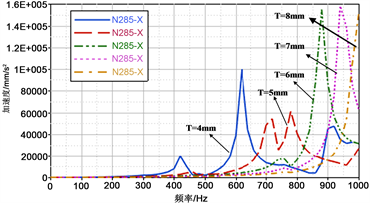(b)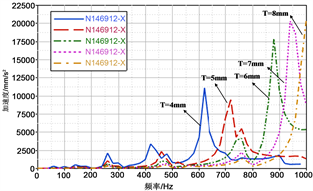(c)

Figure 7. Response of the corresponding position under different stiffness of the cable supports. (a) End of the rod; (b) Balancing weight; (c) Shift stay

5. 结论

1) 在合适的范围内尽量增加整个系统的阻尼，有利于减小二次冲击的峰值。可考虑在拉索支架安装座处添加阻尼橡胶垫。

2) 增加换挡拉索支架的刚度，有利于减小产生的低频成分的二次冲击的峰值，同时提高产生共振的固有频率。可考虑适当增加拉索支架的厚度；改变拉索支架加强筋的结构，以增大刚度。

3) 适当减小换挡拉索接头刚度，有利于增大变形，吸收更多的二次冲击能量。可考虑对换挡拉索接头橡胶进行镂空设计。

NOTES

*通讯作者。

 方向进, 乔湘鹤. 变速器挂挡二次冲击产生原因及改进方案[J]. 汽车零部件, 2016(7): 77-79.

 徐辉辉, 毛祖棋, 万水平, 黄召明. 浅析定位座对手动变速箱二次冲击的影响[J]. 江西化工, 2016(5): 109-112.

 刘荔斌, 张银超, 许长龙. 乘用车手动变速器换挡二次冲击改善策略[J]. 汽车工程学报, 2018, 8(1): 61-65.

 李晓春, 褚超美, 缪国. 汽车同步器换挡二次冲击的动态仿真[J]. 汽车工程, 2014, 36(12): 1498-1502.

 焦红莲, 王玲, 孙晋松. 某手动变速箱换挡性能分析及改进策略[J]. 机械制造, 2015, 44(6): 79-82.

 赵宗祥, 李健. 基于ANSYS软轴式换挡操纵机构的有限元分析[J]. 机械研究与应用, 2016, 29(6): 1-6.

 石晓辉, 苏洪, 张志刚, 李尚. 汽车同步器锁止性能试验研究[J]. 机械设计与制造, 2017(3): 85-88.

 池会强, 褚超美, 缪国, 顾放. 同步器性能参数对同步时间的影响分析研究[J]. 机械设计与制造, 2016(2): 138-144.

 王伟. 某手动变速器动态二次冲击的分析及优化[J]. 测试试验, 2019(6): 108-110.

 周志强, 孙宝群, 汪韶杰, 周友. 某手动变速器仿真分析与优化研究[J]. 农业装备与车辆工程, 2019, 57(3): 49-52.

 傅志方, 华宏星. 模态分析理论与应用[M]. 上海: 上海交通大学出版社, 2000: 50-86.

 杨景义, 王信义. 试验模态分析[M]. 北京: 北京理工大学出版社, 1990: 117-158.

Top